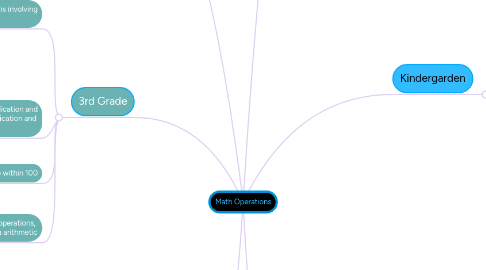# Math Operations

Get Started. It's FreeMath Operations## 1. Kindergarden

### 1.1. understanding addition as putting together and understanding subtraction as taking away

1.1.1. Represent addition and subtraction with objects, fingers, mental images, drawings, sounds, acting out situations, verbal explanations, expressions, or equations

1.1.2. Solve addition and subtraction word problems, and add and subtract within 10 by using objects or drawings to represent the problem

1.1.3. Decompose numbers less than or equal to 10 into pairs in more than one way  by using objects or drawings, and record each decomposition by a drawing or equation      (5 = 2 + 3 and 5 = 4 + 1)

1.1.4. For any number from 1 to 9, find the number that makes 10 when added to the given number by using objects or drawings, and record the answer with a drawing or equation

1.1.5. Fluently add and subtract within 5

### 2.1. represent and solve problems involving addition and subtraction

2.1.1. Use addition and subtraction within 20 to solve word problems involving situations of adding to, taking from, putting together, taking apart, and comparing, with unknowns in all positions, by using objects, drawings, and equations with a symbol for the unknown number to represent the problem

2.1.2. Solve word problems that call for addition of three whole numbers whose sum is less than or equal to 20, by using objects, drawings, and equations with a symbol for the unknown number to represent the problem

### 2.2. Understand and apply properties of operations and the relationship between addition and subtraction

2.2.1. Apply properties of operations as strategies to add and subtract (associative and commutative properties)

2.2.2. Understand subtraction as an unknown-addend problem. For example, subtract 10 - 8 by finding the number that makes 10 when added to 8

### 2.3. Add and subtract within 20

2.3.1. Relate counting to addition and subtraction (counting on 2 to add 2)

2.3.2. Add and subtract within 20, demonstrating fluency for addition and subtraction within 10. Use strategies such as counting on; making ten (8 + 6 = 8 + 2 + 4 = 10 + 4 = 14); decomposing a number leading to a ten      (13 - 4 = 13 - 3 - 1 = 10 - 1 = 9);                using the relationship between addition and subtraction (knowing that 8 + 4 = 12, one knows 12 - 8 = 4);                                      and creating equivalent but easier or known sums (adding 6 + 7 by creating the known equivalent 6 + 6 + 1 = 12 + 1 = 13)

2.3.3. Understand the meaning of the equal sign, and determine if equations involving addition and subtraction are true or false. For example, which of the following equations are true and which are false? 6 = 6, 7 = 8

2.3.4. Determine the unknown whole number in an addition or subtraction equation relating three whole numbers.                                               8 + ? = 11, 5 = _ - 3, 6 + 6 = _.

### 3.1. Represent and solve problems involving addition and subtraction

3.1.1. Use addition and subtraction within 100 to solve one- and two-step word problems involving situations of adding to, taking from, putting together, taking apart, and comparing, with unknowns in all positions, by using drawings and equations with a symbol for the unknown number to represent the problem

### 3.2. Add and subtract within 20

3.2.1. Fluently add and subtract within 20 using mental strategies. By end of Grade 2, know from memory all sums of two one-digit numbers

### 3.3. Work with equal groups of objects to gain foundations of multiplication

3.3.1. Determine whether a group of objects (up to 20) has an odd or even number of members

3.3.2. Use addition to find the total number of objects arranged in rectangular arrays with up to 5 rows and up to 5 columns

### 4.1. Represent and solve problems involving multiplication and division

4.1.1. multiplication of whole numbers

4.1.2. division of whole numbers

4.1.3. use multiplication to solve word problems by using drawings and equations with a symbol for the unknown number to represent the problem

4.1.4. Determine the unknown whole number in a multiplication or division equation relating three whole numbers (8x__=40)

### 4.2. Understand properties of multiplication and the relationship between multiplication and division

4.2.1. Apply properties of operations as strategies to multiply and divide (distributive, commutative, associative)

4.2.2. Understand division as an unknown-factor problem

### 4.3. Multiply and divide within 100

4.3.1. Fluently multiply and divide within 100, using strategies such as the relationship between multiplication and division

### 4.4. Solve problems involving the four operations, and identify and explain patterns in arithmetic

4.4.1. Solve two-step word problems using the four operations

4.4.2. Identify arithmetic patterns and explain them using properties of operations

### 5.1. Use the four operations with whole numbers to solve problems

5.1.1. Interpret a multiplication equation as a comparison

5.1.2. Multiply or divide to solve word problems involving multiplicative comparison

5.1.3. Solve multistep word problems posed with whole numbers and having whole-number answers using the four operations, including problems in which remainders must be interpreted

### 5.2. Gain familiarity with factors and multiplies

5.2.1. Find all factor pairs for a whole number in the range 1-100. Recognize that a whole number is a multiple of each of its factors. Determine whether a given whole number in the range 1-100 is a multiple of a given one-digit number. Determine whether a given whole number in the range 1-100 is prime or composite

### 5.3. Generate and analyze patterns

5.3.1. Generate a number or shape pattern that follows a given rule. Identify apparent features of the pattern that were not explicit in the rule itself